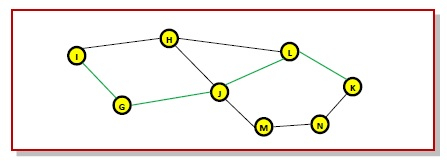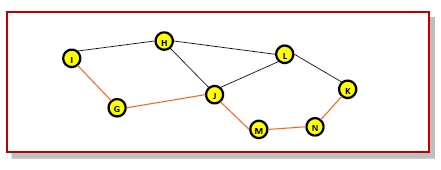# The Optimality Principle in Computer Networks

The optimality principle in computer networks is stated as follows −## Explanation

The purpose of a routing algorithm at a router is to decide which output line an incoming packet should go. The optimal path from a particular router to another may be the least cost path, the least distance path, the least time path, the least hops path or a combination of any of the above.

The optimality principle can be logically proved as follows −

• If a better route could be found between router J and router K, the path from router I to router K via J would be updated via this route. Thus, the optimal path from J to K will again lie on the optimal path from I to K.

## Example

Consider a network of routers, {G, H, I, J, K, L, M, N} as shown in the figure. Let the optimal route from I to K be as shown via the green path, i.e. via the route I-G-J-L-K. According to the optimality principle, the optimal path from J to K with be along the same route, i.e. J-L-K.Now, suppose we find a better route from J to K is found, say along J-M-N-K. Consequently, we will also need to update the optimal route from I to K as I-GJ- M-N-K, since the previous route ceases to be optimal in this situation. This new optimal path is shown line orange lines in the following figure −